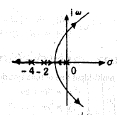# When the plate area of a parallel plate capacitor is increased keeping the capacitor is voltage constant, the force between places

1.  increases

2.  decreases

3.  remains constant

4.  may increase or decrease on the metal making up the plates

4

decreases

Explanation :
No Explanation available for this question

# Two parallel wires separated by a distance d are carrying a DC current I in the same direction. The magnetic field along a line running parallel to these wire and midway between them.

1.  depends upon I

2.   is zero

3.  depends upon d

4.  depends upon the permeability of the medium between the wires

4

is zero

Explanation :
No Explanation available for this question

# Fermi level for an n type semiconductor lies

1.  Near Valence band

2.  Near conduction band

3.  In valence band

4.  In conduction band

4

Near conduction band

Explanation :
No Explanation available for this question

1.

2.

3.

4.

4Explanation :
No Explanation available for this question

# The transfer function of the system shown in the given figure is

1.

2.

3.

4.

4Explanation :
No Explanation available for this question

# The performance specifications for a unity feedback control system having an open-loop transfer function are (ii) Stable closed-loop operation. The value of K, satisfying the above specifications, Is

1.  K>6

2.  6

3.  K>10

4.  None of the above

4

None of the above

Explanation :
No Explanation available for this question

# For the circuit shown in the given figure, assuming ideals diodes, the output waveform V0 will be,

1.

2.

3.

4.

4Explanation :
No Explanation available for this question

# A 24 V, 600 mW Zener diode is to be used for providing a 24 v stabilized supply to a variable load. Assume that for proper Zener action, a minimum of 10 mA must flow through the Zener. If the input voltage is 32 v, what would be the value of R and the maximum load current

1.  320 10 mA

2.  400 ?, 15 mA

3.  400 ?, 10 mA

4.   320 ?, 15 mA

4

320 ?, 15 mA

Explanation :
No Explanation available for this question

# In the series Rc circuit shown in the figure the voltage across C starts increasing when the d. c. source is switched on. The rate of increase of voltage across C at the instant just after the switch is closed (i.e. at t=0+) will be.

1.   zero

2.   infinity

3.  RC

4.  1/ RC

4

1/ RC

Explanation :
No Explanation available for this question

# An ideal voltage source coil charge on ideal capacitor.

1.  in infinite time

2.   exponentially

3.  instantaneously

4.  none of these

4